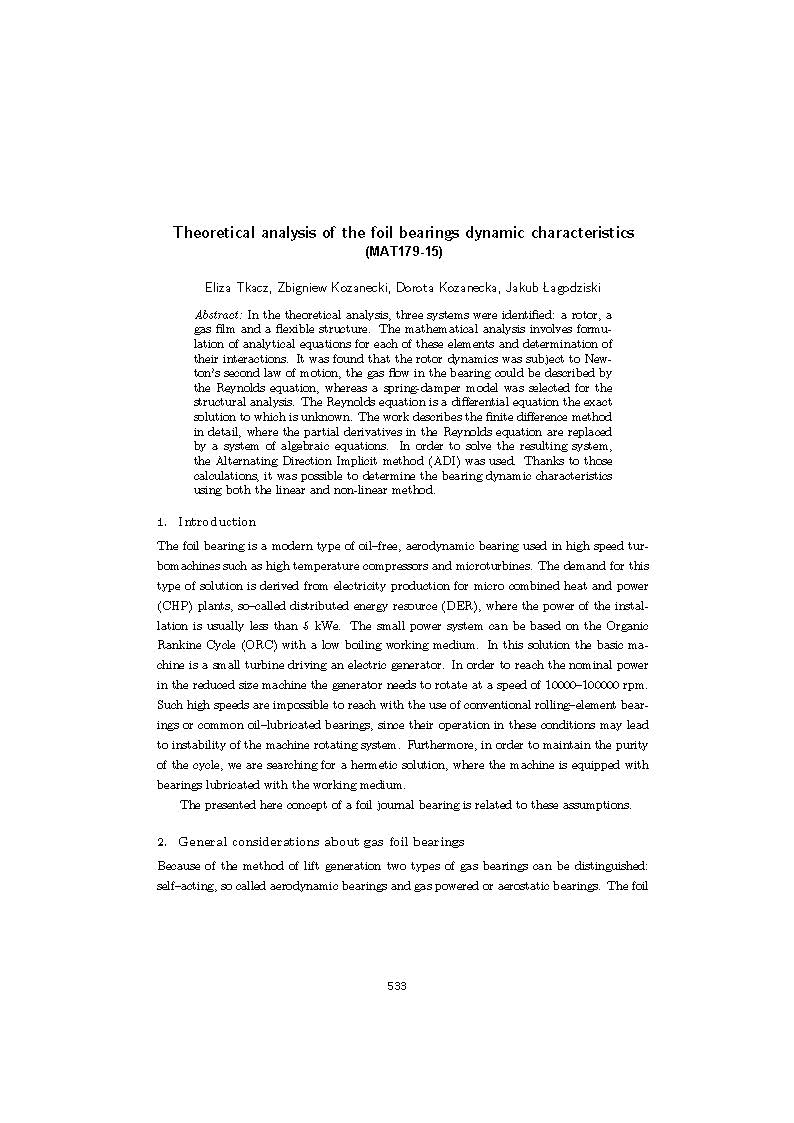# Theoretical analysis of the foil bearings dynamic characteristics.2015

## Wydawca

Politechnika Łódzka. Wydział Mechaniczny. Katedra Automatyki, Biomechaniki i Mechatroniki.

## Abstrakt

In the theoretical analysis, three systems were identified: a rotor, a gas film and a exible structure. The mathematical analysis involves formulation of analytical equations for each of these elements and determination of their interactions. It was found that the rotor dynamics was subject to Newton's second law of motion, the gas flow in the bearing could be described by the Reynolds equation, whereas a spring-damper model was selected for the structural analysis. The Reynolds equation is a differential equation the exact solution to which is unknown. The work describes the finite difference method in detail, where the partial derivatives in the Reynolds equation are replaced by a system of algebraic equations. In order to solve the resulting system, the Alternating Direction Implicit method (ADI) was used. Thanks to those calculations, it was possible to determine the bearing dynamic characteristics using both the linear and non-linear method.

## Słowa kluczowe

systems theory - conference, dynamical systems - conference, teoria systemów, układy dynamiczne, dynamika wirnika, metoda różnic skończonych

## Cytowanie

Dynamical systems : mathematical and numerical approaches ; s. 533-542